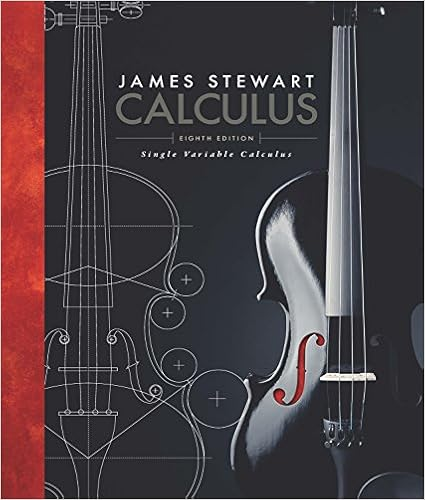# Study Guide - Test 4 (MATH-2212, Spring-2017).pdf -...

• Test Prep
• 7

This preview shows page 1 - 3 out of 7 pages.

##### We have textbook solutions for you!
The document you are viewing contains questions related to this textbook.The document you are viewing contains questions related to this textbook.
Chapter 7 / Exercise 16
Single Variable Calculus
StewartExpert Verified
MATH-2212: Calculus of One Variable II Study Guide for Test 4, Spring 2017 Study Guide for Test 4 It goes without saying that mathematics develops cumulatively, and all subsequent topics usually depend heavily on some (if not all) previous material. This study guide is intended to emphasize the new material learned in this portion of the course. Knowing and understanding everything that has been covered before — in prerequisite courses and earlier in this course — is also crucial for success on this test. Test Contents Section 11.8 – Power Series Section 11.9 – Representation of Functions as Power Series Section 11.10 – Taylor and Maclaurin Series Section 11.11 – Applications of Taylor Polynomials Key Concepts Power series; convergence or divergence of a power series at a point; the radius of convergence and the interval of convergence of a power series. Power series viewed as a function on its interval of convergence; representing functions as power series; term-by-term differentiation and integration of power series. Using power series for approximate numerical integration. Taylor and Maclaurin series of a function; Taylor and Maclaurin polynomials of a function; remainders of Taylor series; representing functions by their Taylor or Maclaurin series. Taylor’s inequality for estimating remainders of Taylor series. Approximating functions and values of functions by Taylor polynomials. Some Important Definitions, Formulas, and Properties Definition. A power series is an expression of the form X n =0 c n x n or X n =0 c n ( x - a ) n with any coefficients c n . More specifically: – 1–
##### We have textbook solutions for you!
The document you are viewing contains questions related to this textbook.The document you are viewing contains questions related to this textbook.
Chapter 7 / Exercise 16
Single Variable Calculus
StewartExpert Verified
MATH-2212: Calculus of One Variable II Study Guide for Test 4, Spring 2017 A power series in x or a power series centered at 0 or a power series about 0 is an expression of the form X n =0 c n x n = c 0 + c 1 x + c 2 x 2 + c 3 x 3 + · · · ; A power series in ( x - a ) or a power series centered at a or a power series about a is an expression of the form X n =0 c n ( x - a ) n = c 0 + c 1 ( x - a ) + c 2 ( x - a ) 2 + c 3 ( x - a ) 3 + · · · . Theorem. Let X n =0 c n x n be a power series centered at 0. If this series converges for x = d , then it also converges for all x satisfying | x | < | d | . If this series diverges for x = d , then it also diverges for all x satisfying | x | > | d | . Theorem. Let X n =0 c n ( x - a ) n be a power series centered at a . Then there exists a value R , 0 R ≤ ∞ , called the radius of convergence of the power series, such that:
•••